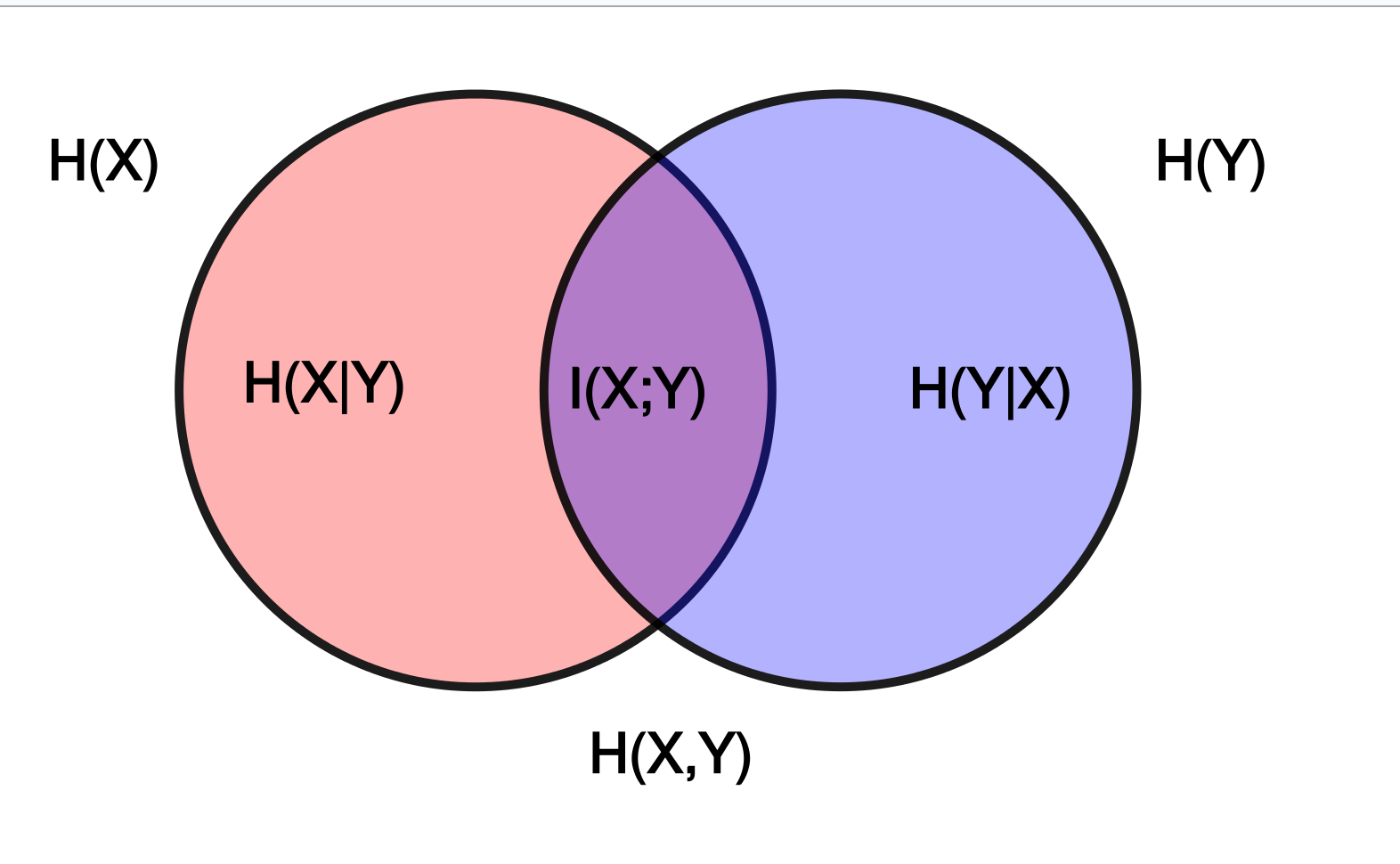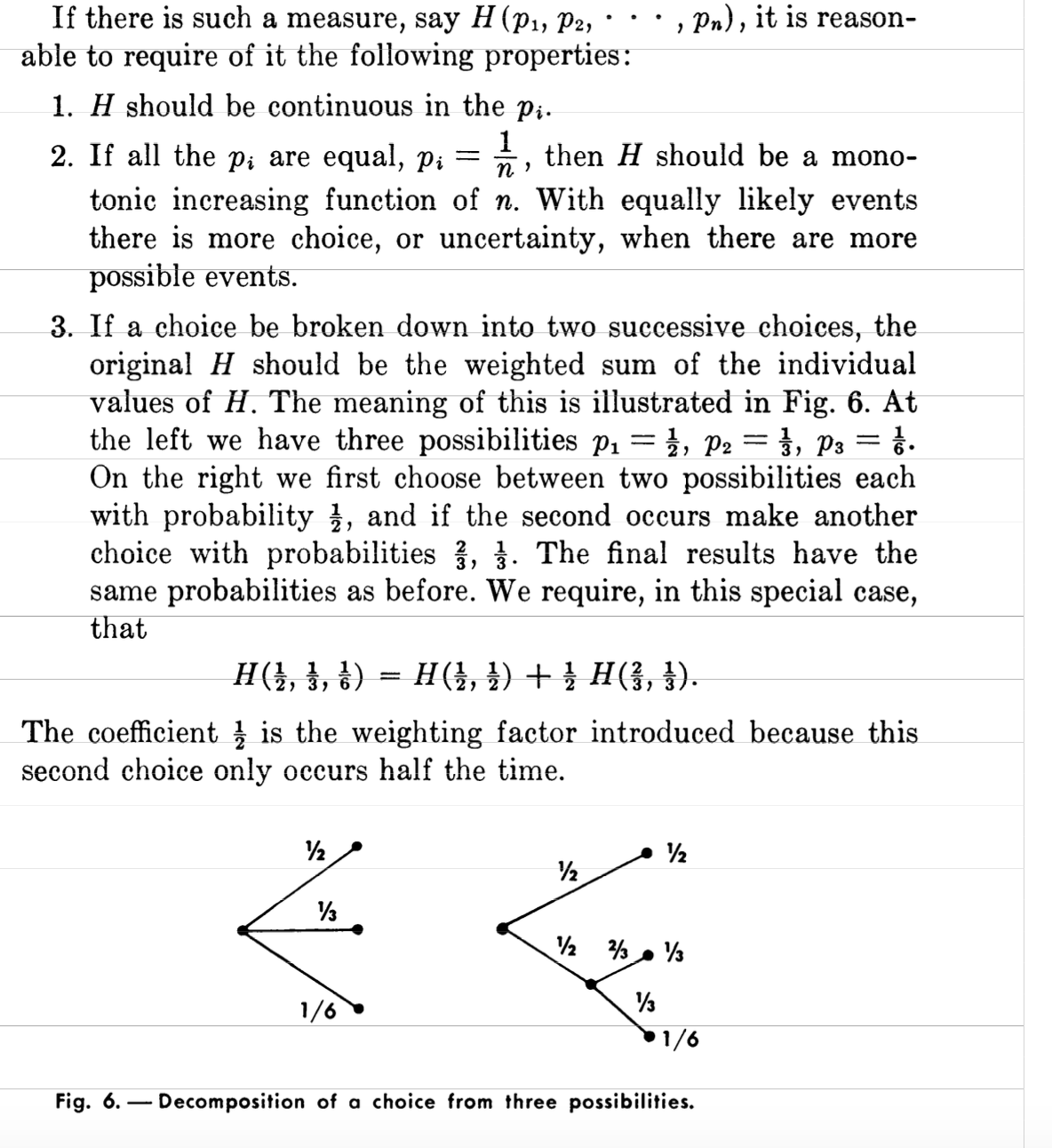记录一下看书收获。


# 1. 什么是熵

熵（Entropy），是一种测量在动力学方面不能做功的能量总数，熵的量度正是能量退化的指标。

1877年，玻尔兹曼发现单一系统中的熵跟构成热力学性质的微观状态数量相关。玻尔兹曼并假设：
S=k*lnΩ



在信息论中，熵是接收的每条消息中包含的信息的平均量，又被称为信息熵、信源熵、平均自信息量。



“My greatest concern was what to call it. I thought of calling it ‘information’,
but the word was overly used, so I decided to call it ‘uncertainty’. When
I discussed it with John von Neumann, he had a better idea. Von Neumann
told me, ‘You should call it entropy, for two reasons: In the first place
your uncertainty function has been used in statistical mechanics under
that name, so it already has a name. In the second place, and more
important, nobody knows what entropy really is, so in a debate you will


# 2. 熵的表示

$$H(X) = E[I(X)] = E[-\ln(P(X))]$$

$$H(X) = - \sum_{i=1}^{m} p(x_i) \cdot \log p(x_i)$$

# 3. 信息熵的来源

柯西函数方程是以下的函数方程：
f(x+y)=f(x)+f(y)



## 3.1 信息量

a)  I(p)随p递增而递减
b)  I(p) ≥ 0
c)  I(1) = 0
d)  I(p1*p2) = I(p1) + I(p2), 即事件独立，具备可加性


$$I(p_1p_2) = I(p_1)+I(p_2)$$

$$p_2I’(p_1p_2) = I’(p_1)$$

$$I’(p_1p_2)+p_1p_2I’’(p_1p_2) = 0$$

$$I’(u)+uI’’(u) = 0$$

$$(u\mapsto uI’(u))’ = 0$$

$$g(x+y)=f(e^{x+y})=f(e^xe^y)=f(e^x)+f(e^y)=g(x)+g(y).$$

f(xy)=f(x)+f(y)特性见：Overview of basic facts about Cauchy functional equation

## 3.2 信息熵

$$H(X) = - \sum {p({x_i})} \log (p({x_i})) ~~~~ (i = 1,2, \ldots ,n)$$

# 4 熵的特性

## 4.1 定义

$${\rm{H(X) = }} - \sum_{x \in X} {p(x)} \ln (p(x)) = - q\ln q - (1 - q)\ln (1 - q)$$

$$H(X,Y) = - \sum_{x \in X,y \in Y} {p(x,y)} \ln (p(x,y)) = - E(\log p(x,y)).$$

\begin{equation}\begin{split} H(Y|X) &=\sum_{x \in X} {p(x)H(Y|X = x)} \\
&=-\sum_x {p(x)} \sum_y {p(y|x)\log p(y|x)} \\
&=-\sum_x {\sum_y {p(x,y)\log p(y|x)} }
\end{split}\end{equation}

$$D({p||q}) = \sum_x {p(x) \log \frac {p(x)}{q(x)}} = E_p(x) \log \frac {p(x)}{q(x)}$$

\begin{equation}\begin{split}
I(X,Y) &= \sum_x \sum_y p(x,y) \log \frac{p(x,y)}{p(x)p(y)} \\
&= D(p(x,y)||(p(x)p(y))) \\
&= E_p(x,y) \log \frac {p(X,Y)} {p(X)p(Y)}
\end{split}\end{equation}

## 4.2 关系

1. H(X,Y)=H(X)+H(Y|X)（链式法则）
即：随机变量X,Y的联合熵等于其中一个随机变量的熵加上另一个随机变量的条件熵。其证明见如下：
\begin{equation}\begin{split}
H(X,Y) &= - \sum_x \sum_y {p(x,y)\log p(x,y)} \\
&= - \sum_x \sum_y {p(x,y)\log p(x)p(y|x)}\\
&= - \sum_x \sum_y {p(x,y)\log p(x)} - \sum_x \sum_y p(x,y)\log p(y|x)\\
&= - \sum_x {p(x)\log p(x)} - \sum_x \sum_y p(x,y)\log p(y|x) \\
&= H(X) + H(Y|X)
\end{split}\end{equation}
2. I(X,Y) = I(Y,X) ,这通过上述定义表达式对称性，可见。
3. I(X,Y) = H(X) -H(Y|X) = H(Y) - H(X|Y), 如下证明：
\begin{equation}\begin{split}
I(X;Y) &= \sum_{x \in X,y \in Y} p(x,y) \log \frac{p(x,y)}{p(x)p(y)}\\
&= \sum p(x,y) \log \frac{p(x|y)}{p(x)} \\
&= - \sum_x \sum_y p(x,y)\log p(x) + \sum_x \sum_y p(x,y)\log p(x|y) \\
& = - \sum_x p(x)\log p(x) - ( - \sum_x \sum_y p(x,y)\log p(x|y) )\\
&= H(X) - H(X|Y)
\end{split}\end{equation}
4. D(P||Q) >=0 即：The relative entropy is non-negative
证明需要用到吉布斯不等式，具体见wikipedia关于这个重要的不等式的意义证明。
若$\sum_1^n p_i =1$ 及 $\sum_1^n q_i = 1$，且$p_i,q_i \in (0,1]$，则有：$-\sum_1^n p_i \log p_i\leq -\sum_1^n p_i\log q_i$，等号成立当且仅当 $p_i=q_i \forall i$
则，上述吉布斯不等式等价于：
$$0\geq \sum_1^np_i\log q_i-\sum_1^n p_i\log p_i=\sum_1^np_i\log(q_i/p_i)=-D(P||Q)$$
得证。
5. I(X,Y) = H(X) -H(Y|X)=H(X)-(H(X,Y)−H(Y)) = H(X)+H(Y)-H(X,Y)
6. I(X,Y) >= 0;见上面I(X,Y)的相对熵表示，同理推出I(X,Y),即The mutual information is positive
7. 也可证 H(X,Y) ≤ H(X)+H(Y), 其实这条可推广至多个变量，即Less than or equal to the sum of individual entropies
8. H(X,Y) ≥ 0, H(X)≥0 joint entropy非负性
9. H(X|Y ) ≤ H(X).Additional information never increases entropy，由3可证，也可根据计算式得证，且仅独立于X,可等。
10. H(X,Y,Z) = H(X) + H(Y|X) + H(Z|XY) ≤ H(X) + H(Y) + H(Z)
11. H(X,Y)≥ max[H(X),H(Y)]，即Greater than individual entropies
12. 还有许多特性，不一一列举。在《通信的数学原理》中香农列举许多特性，并得到许多有趣推论。
最后，引用wikipedia一张图来帮助记忆这几种熵,或参考Joint entropy, Conditional_entropy## 4.3 可加性 - 更好的理解信息熵

1. 怎么去理解可加性？怎么理解 H(X,Y)=H(X)+H(Y|X)链式法则？
其实香农在最初的著作里，用图形象的表达出来了，有助加深理解信息熵的计算不依赖事件先后顺序，也即，假设三个事件P，有p1=1/2,p2=1/3,p3=1/6，我们既可以认为三个时间同时发生，如上图左，也可以是首先有两个事件p1=p2=1/2，然后在第二事件又分为p1=2/3,p2=1/3，所以信息熵H(1/2,1/3,1/6)=H(1/2,1/2)+1/2*H(2/3,1/3)。
一个推导
2. 《数学之美》里，吴军也曾分别举了猜球队冠军、骰子猜概率、王小波的例子形象化信息熵
3. 有个说法是思考如下问题：假设一共有N个球，扔到k个桶中。但这些桶大小是不同的。假设扔到第i个桶里的概率是pi，即第i个桶里有ni个球，那么一共有多少种不同分法（状态数）？
得到结果后将其联想到玻尔兹曼的熵模型。（这个问题的解法其实可以关联到欧拉创造的神奇的伽马函数，这里不展开了）。
4. 为什么要创造信息熵这个概念？其实对应物理／数学模型最大熵的概念

The principle of maximum entropy states that, subject to precisely stated prior data
(such as a proposition that expresses testable information), the probability distribution
which best represents the current state of knowledge is the one with largest entropy.
Another way of stating this: Take a precisely stated prior data or testable information
about a probability distribution function. Consider the set of all trial probability distributions
that would encode the prior data. Of those, one with maximal information entropy is the proper distribution, according to this principle
这很符合人们在解决某系问题时通用的模式，即在已知部分事实的前提下，对未知分布最合理的推断就是符合已知事实的最不确定或最随机的推断。

6. 搜索引擎使用的文档相关性，TD-IDF算法，其实可以理解为相对熵的应用：词频在整个语料库的分布与词频在具体文档中分布之间的差异性。
7. 对于孤立理想气体，在麦克斯韦-玻尔兹曼分布下是稳定态，各相均等，此时是最小熵产生状态。同样的，信息系统有最小鉴别信息原理的说法，可以参考大名鼎鼎的H定理，微观推导出热力学第二定律，也是信息熵公式的前身。
事实上，玻尔兹曼公式的另一种等价表述形式正是 $S=-k \sum_1^n p_i \log p_i)$ ， 其中i标记所有可能的微观态，p(i)表示微观态i的出现几率.
统计熵揭示一个孤立系统的倾向于增加混乱程度，但”宇宙是一个孤立系统“严格来说只是个未被验证的假设。还有H公式等，二者简直是太相似了，甚至通信数学原理许多推论，以及最大熵原理最小鉴别信息原理等都是如此相似？这里物理和信息论如此和谐，这是一种自然现象的两种形式吗，还是二者其实是基于同一数学模型所致？要揭开这样的问题可能需要正态分布／泊松分布／二项分布等各种分布即gama函数等现代概率统计学基础去解释了。
8. 也有使用Shannon熵和热力学熵曲解释现代计算机在处理大量信息时，必须解决散热问题的，如Landauer法则。
9. 玻尔兹曼熵处理的是根据运动的热力学分子均衡状态下，熵增。
而香农观点来看，其实把我们看到的信息，比如一段文字，看成是气体分子，不同的是这里的“气体分子”是比玻尔兹曼的要规则，也即熵小一些。从数学角度去理解：熵就是系统各状态自信息量的数学期望
信息熵或者说熵，或者不论是维纳还是香农的信息论，可以说，比如许多熵减的系统／过程都可以用熵解释，当然这么讲可能不是很有用。
10. 最后，你想过信息论可以解释堆排序为何比快排慢吗？
heapsort和quicksort排序耗时都是O(n*logn), 但为什么quicksort表现会更好？非常建议看看MacKay的Heapsort, Quicksort, and Entropy，【如果被墙，可以看这里】不同于常见的思考问题角度，这篇文章里作者神奇的使用了信息论来解释，每次比较，quicksort获得有用信息的概率比heapsort均等。信息论还可以解释排序极限为何是O(nlogn)
不过这里应该用“阐释”比较好，公式化的论证见TAOCP。
思考下：Java 7偏后版本引入了里面性能被证明更好的TimSort的快速排序(不要再认为是课本里说的二分快排了)，是不是也可以用信息论解释？（TimSort的争议

# 5 最大熵模型

## 5.1 交叉熵Cross entropy)

$$H(p,q) = E_p[-\log q] = -\sum_1^m p(x_i) \log{q(x_i)}$$

\begin{equation}\begin{split} H(p,q) &= -\sum_x p(x) \log q(x) \\
&= -\sum_x p(x) \log \frac{q(x)}{p(x)}p(x)\\
&= -\sum_x p(x) \log p(x) -\sum_x p(x) \log \frac{q(x)}{p(x)}\\
&= H(p)+ D(p||q)
\end{split}\end{equation}

$$\tilde{P}(X=x,Y=y)=\frac{count(X=x,Y=y)}{N}$$
$$\tilde{P}(X=x) = \frac{count(X=x)}{N}$$

$$f(n) = \begin{cases} 1, & \text{if x,y满足某一事实} \\ 0, & \text{else} \\ \end{cases}$$

$$E_{\tilde{P}}(f) = \sum_{ x,y} \tilde{P}(x,y) f(x,y)$$

$$E_P(f) = \sum_{ x,y} {P(x,y) f(x,y)}$$

, p(x)未知，但是我们此时可以使用$\tilde{P}(x)$进行近似,也就是最终有:
$$E_P(f) = \sum_{x,y} P(y|x) \tilde{P}(x) f(x,y)$$

$$\sum_{x,y} \tilde{P}(x,y) f(x,y) = \sum_{x,y} P(y|x) \tilde{P}(x) f(x,y)$$

$$C= {P|E_{\tilde{P}}(f) = E_{P}(f),I=1,2,3..n}$$

$$H(P)= - \sum_{x,y} \tilde{P}(x) P(y|x) log P(y|x)$$

\begin{align}
\underset{P \in C}{max} &\quad H(P) = - \sum_{x,y} \tilde{P}(x) P(y|x) \text{log} P(y|x) \\
st. &\quad E_{P}(f) = E_{\tilde{P}}(f),I=1,2,3..n \\
\end{align}

1. wikipedia
History of entropy
Entropy_(information_theory))
熵 (信息论))
2. 高等数学第六版 同济版
3. 《统计学习方法》.李航著
4. The Mathematical Theory of Communication. by Claude E.Shannon and Warren Weaver